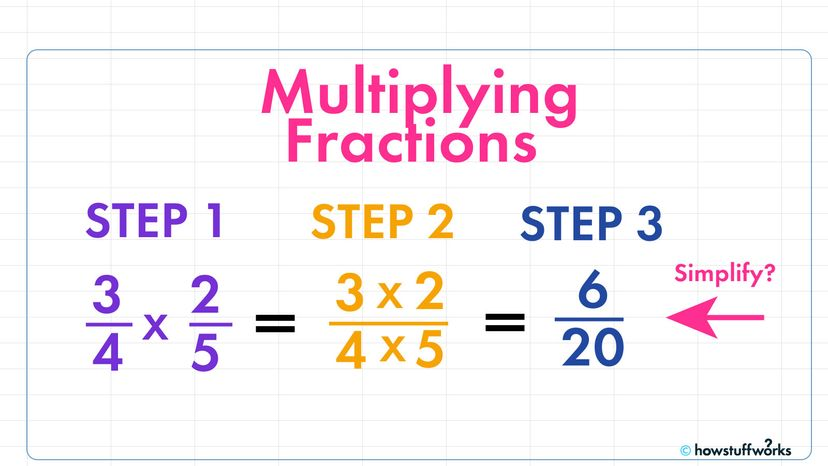# How to Multiply Fractions

By: Jesslyn Shields  |Multiplying fractions is easy if you just follow three simple steps. HowStuffWorks

You might have been in fifth grade the last time you thought about multiplying fractions. But if you're trying to cut a recipe in half or calculate the new price of a sale sweater using fractions, you might need to dig around in the back of your memory for how to do it. Let's refresh:

In every fraction, there's a top number and a bottom number, separated by a short horizontal line. In a proper fraction, the smaller number — called the numerator — will always be on top, while the larger number — the denominator — will be on the bottom. The numerator tells us how many units we have of a whole and the denominator tells us how many units make up the whole. So, in the fraction 1/2, 1 is the numerator and 2 is the denominator — there are two units in the whole, but this fraction tells us that we only have one of these units.

Unlike when you are adding or subtracting fractions, you can multiply fractions with different denominators. For instance, it's not a problem to multiply 3/4 x 2/5.

### Step 1

The first step in multiplying two fractions together is to multiply the numerators together.

3 x 2 = 6

### Step 2

The second step is to multiply the denominators together.

4 x 5 = 20

Easy enough! So our fraction now looks like this:

3/4 x 2/5 = 6/20